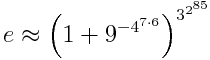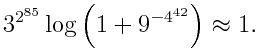# Amazing approximation to e

Here’s an approximation to e by Richard Sabey that uses the digits 1 through 9 and is accurate to over a septillion digits. (A septillion is 1024.)MathWorld says that this approximation is accurate to 18457734525360901453873570 decimal digits. How could you get an idea whether this claim is correct? We could show that the approximation is near e by showing that its logarithm is near 1. That is, we want to showDefine k to be 3^(2^85) and notice that k also equals 9^(4^42). From the power series for log(1 + x) and the fact that the series alternates, we havewhere η is some number between 0 and 1/k. This tells that the error is extremely small because 1/k is extremely small. It also tells us that the approximation underestimates e because its logarithm is slightly less than 1.

Just how small is 1/k? Its log base 10 is around -1.8 × 10^25, so it’s plausible that the approximation is accurate to 10^25 decimal digits. You could tighten this argument up a little and get the exact number of correct digits.

## 7 thoughts on “Amazing approximation to e”

1. Leonid Boytsov

Well, it is not exactly VERY surprising. (1+1/n)^n converges to e. In this case, you have an extremely large n = 3^(2^85)

2. Massimo Ortolano

@vonjd: Because 3^2^85 generates an overflow.

3. …so this result is more of theoretical interest only?

4. John, do you mean that η (eta) should be a little bigger than k? That would make the second term small. If I’m following, then that error term would be something like 1/k–which means that the size of the error has a linear relation to the size of the input k. What would be really fun is some other approximation where this relation is a square or a cube.

5. Joseph

@vonjd For this specific result, probably. If you’re using it for numerics (except perhaps for arbitrary precision perhaps) you’re better off just loading a pre-defined bit pattern which is accurate to whatever precision you want. (And possibly also for arbitrary precision).

For analytic purposes, you just keep e symbolic.

However, the method of finding the number of digits something is valid to (though perhaps in bits instead of decimal) is more generally useful.

IMHO. :)

6. GlennF

Even more amazing if you add (15-14)/(11+13-10-12) to the exponent (It will roughly double the number of digits)